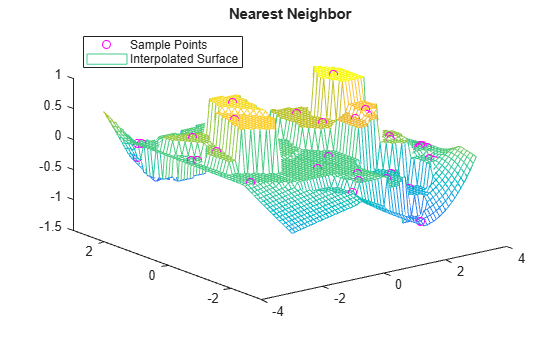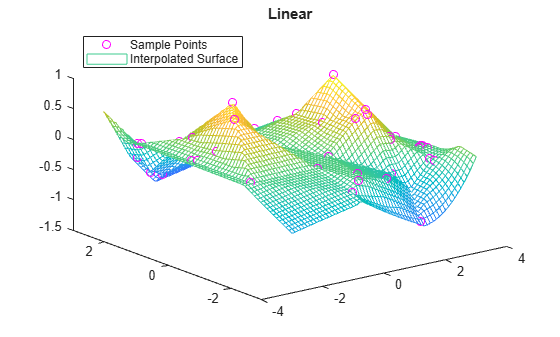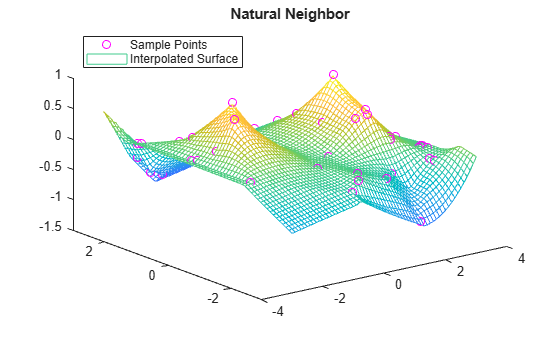# scatteredInterpolant

## 创建对象

### 语法

``F = scatteredInterpolant``
``F = scatteredInterpolant(x,y,v)``
``F = scatteredInterpolant(x,y,z,v)``
``F = scatteredInterpolant(P,v)``
``F = scatteredInterpolant(___,Method)``
``F = scatteredInterpolant(___,Method,ExtrapolationMethod)``

### 说明

``F = scatteredInterpolant` 创建一个空的散点数据插值对象。`

``F = scatteredInterpolant(x,y,v)` 创建一个拟合 v = F(x,y) 形式的曲面的插值。向量 `x` 和 `y` 指定样本点的 `(x,y)` 坐标。`v` 是一个包含与点 `(x,y)` 关联的样本值的向量。`

``F = scatteredInterpolant(x,y,z,v)` 创建一个 v = F(x,y,z) 形式的三维插值。`

``F = scatteredInterpolant(P,v)` 以数组形式指定样本点坐标。`P` 的行包含 `v` 中值的 (x, y) 或 (x, y, z) 坐标。`

``F = scatteredInterpolant(___,Method)` 指定插值方法：`'nearest'`、`'linear'` 或 `'natural'`。在前三个语法中的任意一个中指定 `Method` 作为最后一个输入参数。`

``F = scatteredInterpolant(___,Method,ExtrapolationMethod)` 指定内插和外插方法。在前三个语法的任意一个中同时传递 `Method` 和 `ExtrapolationMethod` 作为最后两个输入参数。`Method` 可以是 `'nearest'`、`'linear'` 或 `'natural'`。`ExtrapolationMethod` 可以是 `'nearest'`、`'linear'` 或 `'none'`。`

### 输入参数

`'linear'`（默认值）

C0
`'nearest'`

`'natural'`

C1（样本点处除外）

ExtrapolationMethod说明
`'linear'`

`'nearest'`

`'none'`

## 用途

```Vq = F(Pq) Vq = F(Xq,Yq) Vq = F(Xq,Yq,Zq) Vq = F({xq,yq}) Vq = F({xq,yq,zq}) ```

• `Vq = F(Pq)` 在矩阵 `Pq` 中指定查询点。`Pq` 中的每行都包含查询点的坐标。

• `Vq = F(Xq,Yq)``Vq = F(Xq,Yq,Zq)` 将查询点指定为两个或三个大小相等的矩阵。

• `Vq = F({xq,yq})``Vq = F({xq,yq,zq})` 将查询点指定为网格向量。使用此语法可在您要查询大型点网格时节省内存。

## 示例

```t = linspace(3/4*pi,2*pi,50)'; x = [3*cos(t); 2*cos(t); 0.7*cos(t)]; y = [3*sin(t); 2*sin(t); 0.7*sin(t)]; v = repelem([-0.5; 1.5; 2],length(t));```

`F = scatteredInterpolant(x,y,v);`

```tq = linspace(3/4*pi+0.2,2*pi-0.2,40)'; xq = [2.8*cos(tq); 1.7*cos(tq); cos(tq)]; yq = [2.8*sin(tq); 1.7*sin(tq); sin(tq)]; vq = F(xq,yq);```

```plot3(x,y,v,'.',xq,yq,vq,'.'), grid on title('Linear Interpolation') xlabel('x'), ylabel('y'), zlabel('Values') legend('Sample data','Interpolated query data','Location','Best')``````rng default; P = -2.5 + 5*rand([200 3]); v = sin(P(:,1).^2 + P(:,2).^2 + P(:,3).^2)./(P(:,1).^2+P(:,2).^2+P(:,3).^2);```

`F = scatteredInterpolant(P,v);`

```[xq,yq,zq] = meshgrid(-2:0.25:2); vq = F(xq,yq,zq);```

```xslice = [-.5,1,2]; yslice = [0,2]; zslice = [-2,0]; slice(xq,yq,zq,vq,xslice,yslice,zslice)``````rng('default') x = -2.5 + 5*rand([50 1]); y = -2.5 + 5*rand([50 1]); v = x.*exp(-x.^2-y.^2);```

`F = scatteredInterpolant(x,y,v)`
```F = scatteredInterpolant with properties: Points: [50x2 double] Values: [50x1 double] Method: 'linear' ExtrapolationMethod: 'linear' ```

`(1.40,1.90)` 处计算插值。

`F(1.40,1.90)`
```ans = 0.0069 ```

```vnew = x.^2 + y.^2; F.Values = vnew; F(1.40,1.90)```
```ans = 5.6491 ```

```P = -2.5 + 5*rand(200,3); P(197:200,:) = repmat(P(196,:),4,1);```

`V = rand(size(P,1),1);`

`[V_unique,P_unique] = groupsummary(V,P,@max);`

`P_unique = [P_unique{:}];`

`I = scatteredInterpolant(P_unique,V_unique);`

```x = -3 + 6*rand(50,1); y = -3 + 6*rand(50,1); v = sin(x).^4 .* cos(y);```

```F = scatteredInterpolant(x,y,v); [xq,yq] = meshgrid(-3:0.1:3);```

```F.Method = 'nearest'; vq1 = F(xq,yq); plot3(x,y,v,'mo') hold on mesh(xq,yq,vq1) title('Nearest Neighbor') legend('Sample Points','Interpolated Surface','Location','NorthWest')``````F.Method = 'linear'; vq2 = F(xq,yq); figure plot3(x,y,v,'mo') hold on mesh(xq,yq,vq2) title('Linear') legend('Sample Points','Interpolated Surface','Location','NorthWest')``````F.Method = 'natural'; vq3 = F(xq,yq); figure plot3(x,y,v,'mo') hold on mesh(xq,yq,vq3) title('Natural Neighbor') legend('Sample Points','Interpolated Surface','Location','NorthWest')``````figure plot3(x,y,v,'mo') hold on mesh(xq,yq,sin(xq).^4 .* cos(yq)) title('Exact Solution') legend('Sample Points','Exact Surface','Location','NorthWest')``````rng('default') P = -2.5 + 5*rand([200 2]); x = P(:,1); y = P(:,2); v = x.*exp(-x.^2-y.^2);```

`F = scatteredInterpolant(P,v,'linear','nearest')`
```F = scatteredInterpolant with properties: Points: [200x2 double] Values: [200x1 double] Method: 'linear' ExtrapolationMethod: 'nearest' ```

`vq = F(3.0,-1.5)`
```vq = 0.0029 ```

```F.ExtrapolationMethod = 'none'; vq = F(3.0,-1.5)```
```vq = NaN ```

## 提示

• 计算多组不同查询点处的 `scatteredInterpolant` 对象 `F` 的值比使用函数 `griddata``griddatan` 单独计算插值的速度更快。例如：

```% Fast to create interpolant F and evaluate multiple times F = scatteredInterpolant(X,Y,V) v1 = F(Xq1,Yq1) v2 = F(Xq2,Yq2) % Slower to compute interpolations separately using griddata v1 = griddata(X,Y,V,Xq1,Yq1) v2 = griddata(X,Y,V,Xq2,Yq2) ```
• 要更改插值样本值或插值方法，更新插值对象 `F` 的属性比创建新 `scatteredInterpolant` 对象的效率更高。当您更新 `Values``Method` 时，输入数据的基础 Delaunay 三角剖分不会更改，因此您可以快速计算新结果。

• 使用 `scatteredInterpolant` 进行的散点数据插值使用数据的 Delaunay 三角剖分，因此对采样点 `x``y``z``P` 中的缩放问题非常敏感。出现这种情况时，您可以使用 `normalize` 重新缩放数据并改进结果。有关详细信息，请参阅对不同量级的数据进行归一化

## 算法

`scatteredInterpolant` 使用散点样本点的 Delaunay 三角剖分执行插值 

 Amidror, Isaac. “Scattered data interpolation methods for electronic imaging systems: a survey.” Journal of Electronic Imaging. Vol. 11, No. 2, April 2002, pp. 157–176.